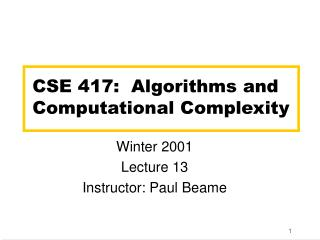Download PresentationCSE 417: Algorithms and Computational ComplexityCSE 417: Algorithms and Computational Complexity - PowerPoint PPT Presentation

Download PresentationCSE 417: Algorithms and Computational Complexity
An Image/Link below is provided (as is) to download presentation

Download Policy: Content on the Website is provided to you AS IS for your information and personal use and may not be sold / licensed / shared on other websites without getting consent from its author. While downloading, if for some reason you are not able to download a presentation, the publisher may have deleted the file from their server.

- - - - - - - - - - - - - - - - - - - - - - - - - - - E N D - - - - - - - - - - - - - - - - - - - - - - - - - - -
Presentation Transcript

1. CSE 417: Algorithms and Computational Complexity Winter 2001 Lecture 13 Instructor: Paul Beame

2. All-pairs shortest paths • If no negative-weight edges and sparse graphs run Dijsktra from each vertex O(nm log n) • What about other cases?

3. w v interior vertices Floyd’s Algorithm Idea • Interior vertices in a path • Dijsktra’s Algorithm • at each step always computed shortest paths that only had interior vertices from a set S at each step • Floyd’s Algorithm • slowly add interior vertices on fixed schedule rather than depending on the graph

4. Floyd’s Algorithm • Vertices V={v1,...,vn} • Let Dk[i,j] = length of shortest path from vi to vj that only allows {v1,...,vk} as interior vertices • Note • D0[i,j] = weight of edge (vi,vj) if(vi,vj)Eif(vi,vj)E • Dn[i,j] = length of shortest path from vito vj

5. vi vertices from v1,...,vk vi vi vi vertices from v1,...,vk-1 Floyd’s Algorithm • Computing Dk[i,j] Case 1:vk not used Dk[i,j] =Dk-1[i,j]

6. vj vi Floyd’s Algorithm Case 2:vk used vertices from v1,...,vk-1 vk vertices from v1,...,vk-1 vertices from v1,...,vk-1

7. vj vi Floyd’s Algorithm Case 2:vk used vertices from v1,...,vk-1 not on shortest path since no negative cycles vk vertices from v1,...,vk-1 vertices from v1,...,vk-1

8. vj vi Floyd’s Algorithm Case 2:vk used Dk[i,j] = Dk-1[i,k] + Dk-1[k,j] vk vertices from v1,...,vk-1 vertices from v1,...,vk-1

9. Floyd’s Algorithm O(n3) time O(n2) storage by maintaining array for last two values of k • Floyd(G) D0weight matrix of G for k=1 to n dofor i=1 to n dofor i=1 to n do Dk[i,j] min{ Dk-1[i,j], Dk-1[i,k]+Dk-1[k,j]} endforendforendfor

10. Multiplying Faster • On the first HW you analyzed our usual algorithm for multiplying numbers • Q(n2) time • We can do better! • We’ll describe the basic ideas by multiplying polynomials rather than integers • Advantage is we don’t get confused by worrying about carries at first

11. Note on Polynomials • These are just formal sequences of coefficients so when we show something multiplied by xk it just means shifted k places to the left

12. Polynomial Multiplication • Given: • Degree n-1 polynomials P and Q • P = an-1xn-1+an-2xn-2+ … +a2 x2+a1 x +a0 • Q = bn-1xn-1+bn-2xn-2+ … +b2 x2+b1 x +b0 • Compute: • Degree 2n-2 Polynomial PQ • PQ =an-1bn-1x2n-2+ (an-1bn-2+ an-2bn-1) x2n-3+ ... + (an-1bi+1+an-2bi+2+...+ai+1bn-1) xn+i + ... + a0b0 • Obvious Algorithm: • Compute all aibj and collect terms • Q (n2)time

13. Naive Divide and Conquer • Assume n is a power of 2 • P = (an-1xn/2-1 + an-2xn/2-2 + … + an/2) xn/2 + (an/2-1 xn/2-1 + ... + a2 x2 + a1 x + a0) = P1xn/2 + P0 • Q = Q1xn/2+ Q0 • PQ = (P1xn/2+P0)(Q1xn/2+Q0) = P1Q1xn+(P1Q0+P0Q1)xn/2+P0Q0 • 4 sub-problems of size n/2 + plus linear combining • T(n)=4T(n/2)+cn • Solution T(n) = O(n2)

14. Karatsuba’s Algorithm • A better way to compute the terms • Compute • P0Q0 • P1Q1 • (P1+P0)(Q1+Q0) which is P1Q1+P1Q0+P0Q1+P0Q0 • Then • P0Q1+P1Q0 = (P1+P0)(Q1+Q0) - P0Q0- P1Q1 • 3 sub-problems of size n/2 plus O(n) work • T(n) = 3 T(n/2) + cn • T(n) = O(na) where a = log23 = 1.59...